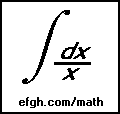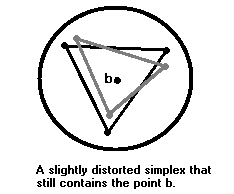Approximate Matrix Inverses and the Condition Number

June 9, 2001

About 32 years ago, I published what some have described as an "elegant result" on the matrix condition number. It states that a nonsingular matrix has widely different approximate right and left inverses if, and only if, it is ill-conditioned. However, I did not publish a proof. The proof is not particularly difficult. It is published for the first time here.

All of the following results deal with finite-dimensional real vector spaces. Vectors are conventionally represented by COLUMN vectors. All vectors are the same size and all matrices are square real matrices with the same number of rows as the vectors.

There are standard extensions to finite-dimensional complex vector spaces, but some results are not true in the infinite-dimensional case.

Definition. A vector norm is a real-valued function ||x|| of a vector x such that for all vectors x and y and all scalars c:

1. ||x|| >= 0 with equality if, and only if, x is zero.
2. ||cx|| = |c| ||x||.
3. ||x + y|| <= ||x|| + ||y||.

One example of a vector norm is the Euclidean norm:

||x|| = sqrt(xT x).

Another one is the "maximum norm":

||x|| = max (|x1|, |x2|, ..., |xn|),

where x1, x2, ..., xn are the entries in the vector x.

Definition. The matrix norm corresponding to a vector norm is the real-valued function ||A|| of the matrix A defined by

||A|| = sup ||x|| = 1 ||Ax||.

Compactness arguments show that the supremum always exists and is attained; hence there is always at least one nonzero vector x for which

||Ax|| = ||A|| ||x||.
Alternatively ||A|| could be defined as the number such that
||Ax|| <= ||A|| ||x||,
for all x, with equality for at least one nonzero x. It is fairly easy to prove that if A and B are matrices and c is a scalar,
1. ||A|| >= 0 with equality if, and only if, A = 0.
2. ||cA|| = |c| ||A||.
3. ||A + B|| <= ||A|| + ||B||.
4. ||AB|| <= ||A|| ||B||.

Definition. The condition number of a nonsingular matrix A with respect to a matrix norm is

||A|| ||A-1||.

A matrix with a high condition number is said to be ill-conditioned.

The proof of the following proposition requires some convexity theory (see Appendix A).

Proposition. For every vector norm and every pair of vectors x and y, with x nonzero, there is a matrix A such that Ax = y and ||A|| = ||y|| / ||x||.

Proof. The set {z | ||z|| <= ||x||} is bounded and convex and x is on its boundary. Therefore, there is a support hyperplane (sometimes called a tangent hyperplane) at x. Then there is a basis

x, s2 , s3, ..., sn,

in which all vectors except x are parallel to the support hyperplane.

Now let A be the matrix of the linear mapping which carries x into y and every other basis vector into zero; i.e., for any vector w

w = cx + c2s2 + c3s3 + ... + cnsn,

Aw = cy.

Then if c is not zero,

w/c = x + (c2/c)s2 + (c3/c)s3 + ... + (cn/c)sn .

Since w/c is on the support hyperplane, it must lie on the boundary or in the exterior of the set {z | ||z|| <= ||x||}. Hence

||w/c|| >= ||x||,

||w|| >= |c| ||x||.

Hence

||Aw|| / ||w|| = ||cy|| / ||w|| <= (|c| ||y||) / (|c| ||x||) = ||y|| / ||x||.

If c = 0, then ||Aw|| = 0, so the inequality still holds. Moreover, we have equality when w = x. Hence

||A|| = ||y|| / ||x||.

Theorem. For any nonsingular real matrix A and any real matrix B not equal to the inverse of A,

||AB - I|| / ||BA - I|| <= ||A|| ||A-1||,

with equality for some matrix B arbitrarily close to the inverse of A.

Also,

||BA - I|| / ||AB - I|| <= ||A|| ||A-1||,

with equality for some matrix B arbitrarily close to the inverse of A.

Proof. Let D = BA - I. Then the first assertion becomes

||ADA-1|| / ||D|| <= ||A|| ||A-1||,

with equality for some nonzero D. We can make D arbitrarily close to zero by simply scaling it.

Now by (4) above, inequality is easy to prove. To prove the existence of a matrix D for which equality holds, let x and y be nonzero vectors such that

||A-1x|| = ||A-1|| ||x||,

||Ay|| = ||A|| ||y||.

Let D be a matrix such that

D(A-1x) = y,

||D|| = ||y|| / ||A-1x|| .

Then

||ADA-1|| ||x|| >= ||ADA-1x|| = ||Ay|| = ||A|| ||y|| = ||A|| ||A-1x|| ||D|| = ||A|| ||A-1|| ||x|| ||D||,

from which the desired result follows immediately.

The proof of the other assertion is similar.

Appendix A - A Little Convexity Theory

This part was added on October 30, 2006.

A subset C of ℜn (n-dimensional real vector space) is called convex if for every x and y in C, the line segment joining them is also in C, i.e. tx+ (1-t)y is in C for every t between 0 and 1.

It is easily shown that the interior and the closure of a convex set are also convex, and that the intersection of two convex sets is convex.

An m-dimensional simplex of ℜn is the set of all linear combinations of m+1 points x0, x1, x2, ... , xm (called the vertices) with nonnegative coefficients whose sum is 1:

{a0x0 + a1x1 + a2x2 + ... + amxm | a0 + a1 + a2 + ... + am = 1, a0 >= 0, a1 >= 0, a2 >= 0, ..., am >= 0},

and where the vectors x1-x0, x2-x0, ... , xm-x0 are linearly independent.

A one-dimensional simplex is a line segment; a two-dimensional simplex is a triangle and a three-dimensional simplex is a tetrahedron.

It is easy to show that if a convex set contains all the vertices of a simplex, it contains every point of the simplex.

A hyperplane is a translated (n-1)-dimensional subspace of ℜn, i.e., it is of the form {h+s | s in S}, where h is a point in the hyperplane and S is an (n-1)-dimensional subspace S of ℜn. (This representation is not unique, because h can be any point on the hyperplane.) The hyperplane is said to be the hyperplane passing through h and parallel to S. A hyperplane in ℜ1 is a point, a hyperplane in ℜ2 is a straight line, and a hyperplane in ℜ3 is a plane.

Theorem A.1.. A hyperplane in ℜn divides ℜn into two pieces; more precisely, the complement of the hyperplane consists of two disjoint open sets, which are called the two sides of the hyperplane. Moreover, a line segment from one side to the other passes through the hyperplane.

Proof. Suppose the hyperplane passes through h and is parallel to S, and let s1, s2, ..., sn-1 be a basis for S. For any x in ℜn let f(x) be the determinant of the matrix whose columns are s1, s2, ..., sn-1 and x-h. Then f(x)=0 if, and only if, x is on the hyperplane. Since f is continuous, the two sets {x in ℜn | f(x) < 0} and {x in ℜn | f(x) > 0} are the required open sets.

The intermediate value theorem can be used to prove the other part.

Theorem A.2. Given a nonempty convex set C in ℜn and a point x in the exterior of C, there is a hyperplane separating C from x; i.e., x and C lie on opposite sides of the hyperplane.

Proof. Consider a point c in the closure of C closest to x (using the usual Euclidean metric); standard compactness arguments show that such a point must exist. (It can also be shown to be unique, but uniqueness is not required for this proof.)

Since x is in the exterior of C, c is distinct from x. Hence there is an (n-1) dimensional subspace S of ℜn perpendicular to the line segment joining c and x, and there is a hyperplane passing through the midpoint of the line segment and parallel to S.

The points x and c are on opposite sides of the hyperplane.

 Now assume, for purpose of contradiction, that there is a point d in C on the hyperplane or on the same side as x. Since the closure of C is convex, the line segment from d to c lies entirely in the closure of C. The accompanying illustration shows the relationship in a two-dimensional plane containing d, c and x. The angle dcx is acute, so some point on the line segment sufficiently close to c must be closer to x than c, which is a contradiction, because c was the closest point in the closure of C to x.The next theorem relies on the following proposition, which is obvious for convex sets of the kind used here, but is in fact true for all convex sets.

Proposition A.3. Let S be a convex set in ℜn, and let b be a boundary point of S. Then there points in the exterior of S that are arbitrarily close to b.

Proof. Assume, for purpose of contradiction, that some neighborhood of b contains no points in the exterior of S. Hence every point in the neighborhood is either a boundary point or an interior point of S.

 Now construct an n-dimensional simplex inside the neighborhood with b in its interior. The vertices of the simplex must be either points in S or boundary points of S. In either case, there are points arbitrarily close to them that do lie in S. Choose such points to construct a slightly distorted simplex that still includes b.Since S is convex, it contains all points in the distorted simplex. Hence the point b is an interior point of S. This is the desired contradiction.

A hyperplane is called a tangent hyperplane, or a support hyperplane of a set in ℜn at a boundary point if (1) the hyperplane contains the boundary point and (2) all other points in the set which are not on the hyperplane lie on one side of the hyperplane.

Theorem A.4. A convex set in ℜn has at least one tangent hyperplane at every boundary point.

Proof. Let b be a boundary point of a convex set S, and let x1, x21, ... be a sequence of exterior points approaching b. By Theorem A.3 for every xi there is a hyperplane Hi separating b from S. Some subsequence of these hyperplanes converges to the desired tangent hyperplane.

You may ask how hyperplanes can converge. Just associate Hi with the point where it crosses the line joining xi to the closest point in the closure of S and a normalized basis for its subspace S. The coordinates of all these vectors are bounded, so all of them have convergent subsequences.